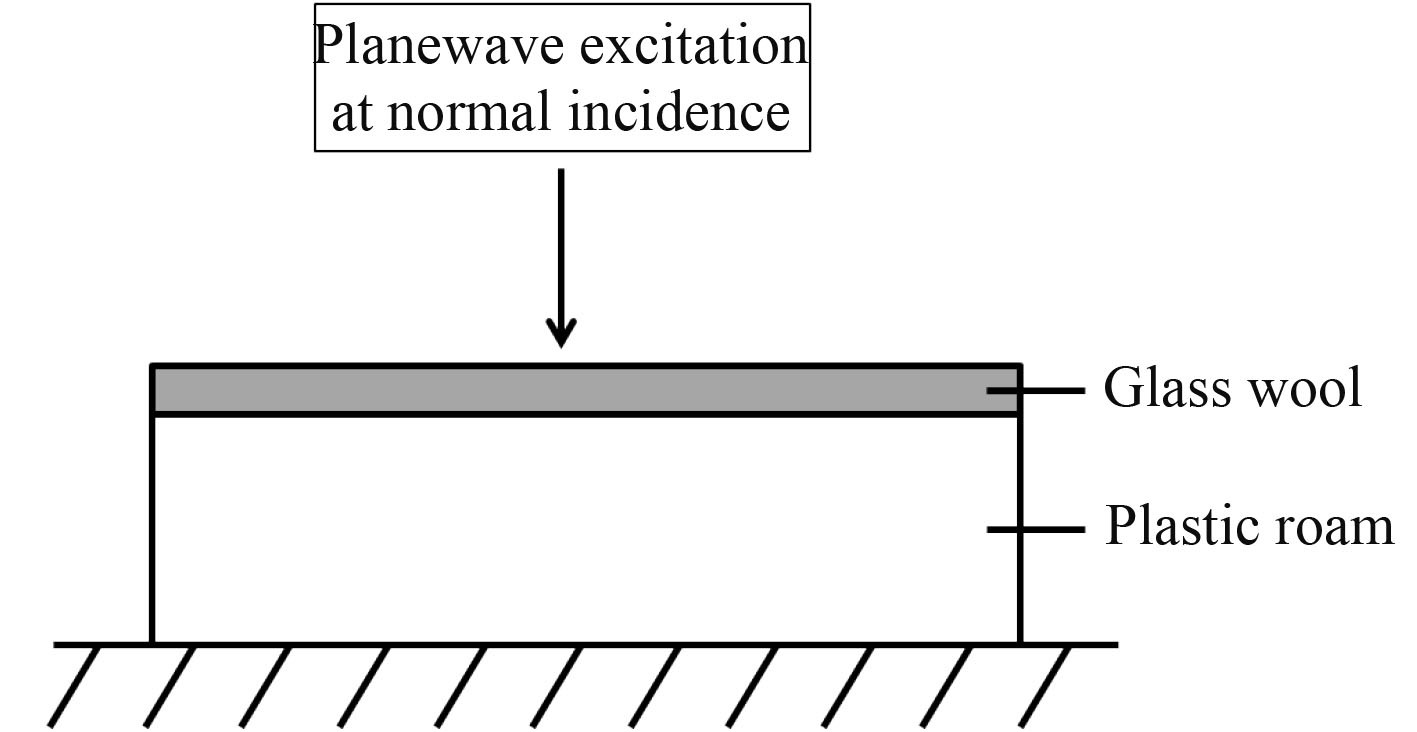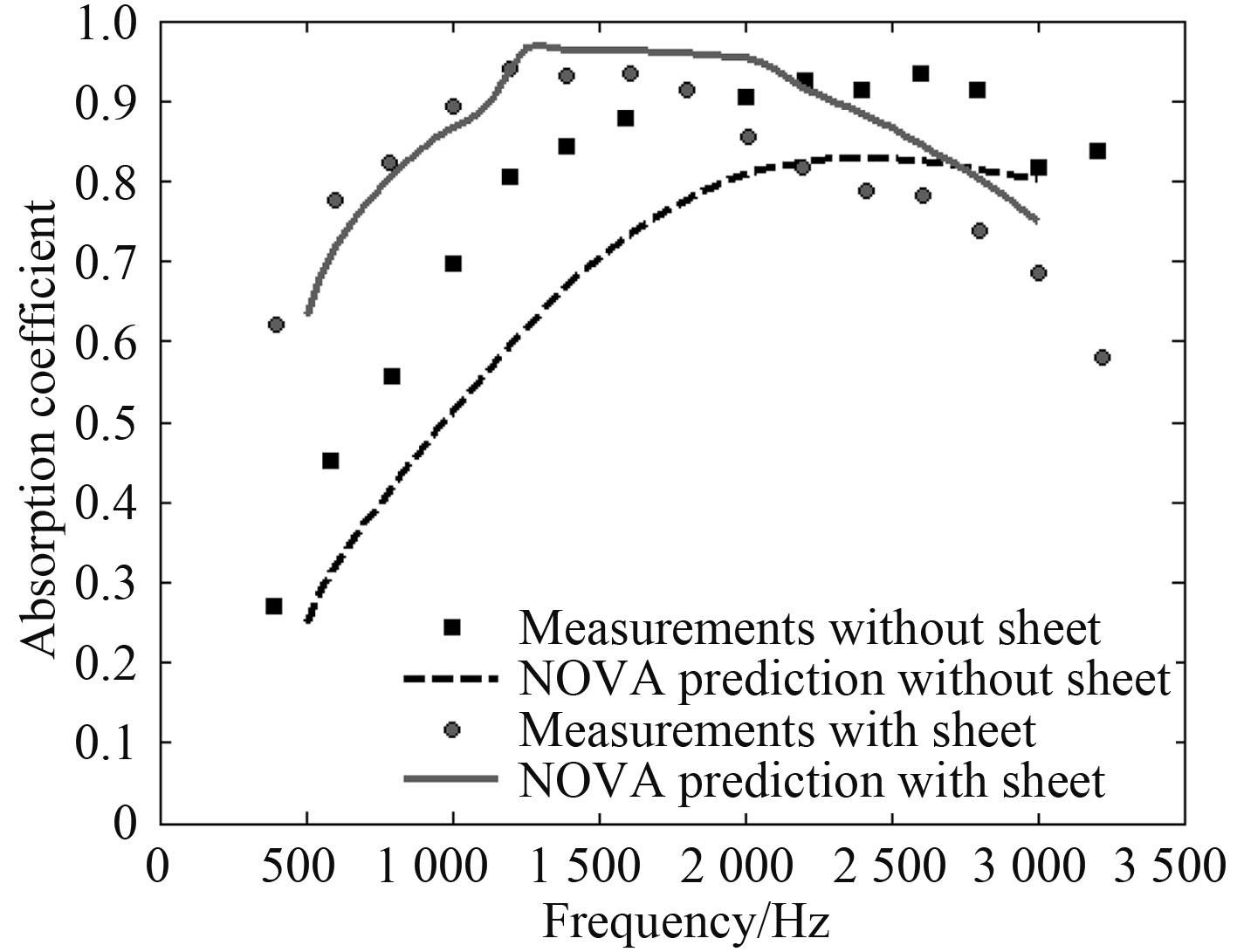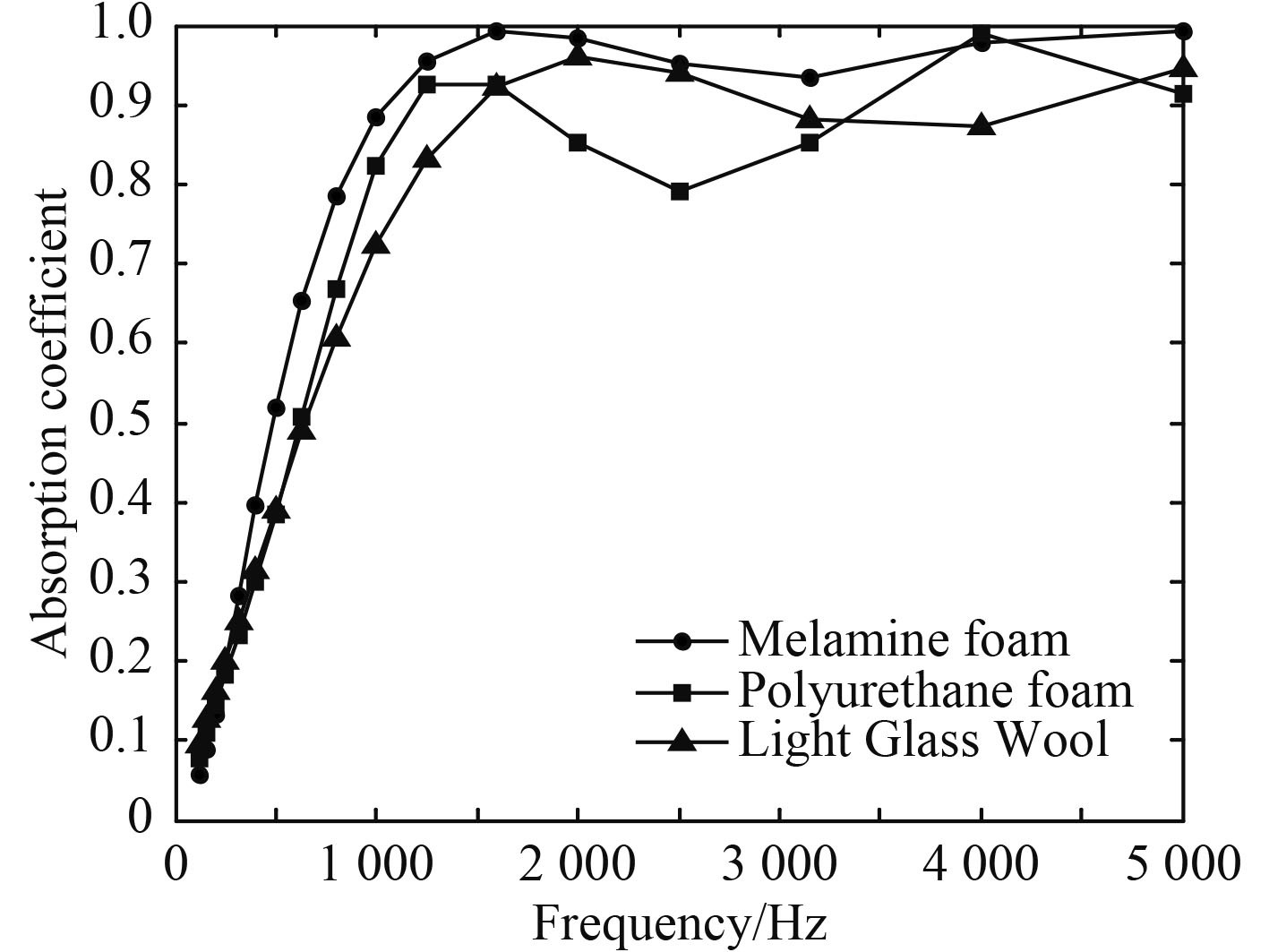﻿ 基于NOVA的多孔材料吸声性能分析及优化
 舰船科学技术2017, Vol. 39Issue (2): 61-64,74PDF

Analysis and optimization for sound absorption performance of porous materials based on NOVA
ZHANG Jin-lan, QIAN Jia-chang, WANG Wen-bo
Wuhan Second Ship Design and Research Institute, Wuhan 430064, China
Abstract: Melamine foam is a kind of excellent porous absorption material. The sound absorption characteristics of melamine foam were analyzed by NOVA. Firstly, the calculated sound absorption coefficient of the porous material was compared with the measured value, which validated the result predicted by NOVA. Then, the absorption coefficient curves of three common porous materials were analyzed. Finally, four parameters, material thickness, density, porosity and thickness of back cavity were taken as the factors. Four levels were selected for each factor. Orthogonal experiment was carried out using L16(45) orthogonal array. The combination of the levels which make the average sound absorption coefficient the highest is found. Through the analysis of variance, it is clear that the thickness of material and back cavity are the main factors affecting the sound absorption performance of melamine foam.
Key words: biot theory     sound absorption coefficient     melamine foam     orthogonal experiment
0 引 言

1 多孔材料声传播理论

 $\sigma _{ij}^s = \left[ {\left( {P - 2N} \right){\theta ^s} + Q{\theta ^f}} \right]{\delta _{ij}} + 2Ne_{ij}^s\text{，}$ (1)
 $\sigma _{ij}^f = \left( { - \phi p} \right){\delta _{ij}} = \left( {Q{\theta ^s} + R{\theta ^f}} \right){\delta _{ij}}\text{。}$ (2)

 $\begin{array}{l} {\delta _{ij}} = 1\;\;\;\;if\;\;\;\;i = j\text{，}\\ {\delta _{ij}} = 0\;\;\;\;if\;\;\;\;i \ne j\text{。} \end{array}$ (3)

PQR 为弹性系数，与多孔材料的结构和属性有关。

 $P = \frac{{\left( {1 - \phi } \right)\left[ {1 - \phi - {K_b}/{K_s}} \right]{K_s} + \phi {K_b}{K_s}/{K_f}}}{{1 - \phi - {K_b}/{K_s} + \phi {K_s}/{K_f}}} + \frac{4}{3}N\text{，}$ (4)
 $Q = \frac{{\left( {1 - \phi - {K_b}/{K_s}} \right)\phi {K_s}}}{{1 - \phi - {K_b}/{K_s} + \phi {K_s}/{K_f}}}\text{，}$ (5)
 $R = \frac{{{\phi ^2}{K_s}}}{{1 - \phi - {K_b}/{K_s} + \phi {K_s}/{K_f}}}\text{。}$ (6)

Allard 对式（4）~ 式（6）进行了简化，对于大多数多孔介质，构成框架的材料可以认为不可压，即Ks 非常大，此时弹性系数可表示为，

 $P = {K_b} + \frac{{{{\left( {1 - \phi } \right)}^2}}}{\phi }{K_f} + \frac{4}{3}N\text{，}$ (7)
 $Q = \left( {1 - \phi } \right){K_f}\text{，}$ (8)
 $R = \phi {K_f}\text{。}$ (9)

 ${K_b} = \frac{{2N\left( {1 + \nu } \right)}}{{3\left( {1 - 2\nu } \right)}}\text{，}$ (10)

 ${K_f} = {P_0}/\left( {1 - \frac{{\gamma - 1}}{{\gamma \alpha '\left( \omega \right)}}} \right)\text{，}$ (11)
 $\gamma = {c_p}/{c_v}\text{，}$ (12)
 $\alpha '\left( \omega \right) = \frac{{8\nu '}}{{j\omega \Lambda {'^2}}}{\left[ {1 + {{\left( {\frac{{\Lambda '}}{4}} \right)}^2}\frac{{j\omega }}{{\nu '}}} \right]^{1/2}} + 1\text{，}$ (13)
 $\nu ' = \frac{\kappa }{{{\rho _0}{c_p}}}\text{。}$ (14)

2 数值计算 2.1 方法验证

NOVA 是一款由 ESI 集团推出的基于传递矩阵法（TMM）的多功能声学预报软件。为验证 NOVA 计算的准确性，首先对一种多孔结构的吸声特性进行预报，并将结果与文献[6,8]中实测值进行对比。该多孔结构主要由泡沫塑料组成，其表面还覆盖着一层很薄的玻璃棉，结构背面为刚性壁，激励声源为垂直平面入射波如图 1 所示，材料参数见表 1图 1 多孔结构横截面示意图 Fig. 1 Porous structure form in cross-section表 1 多孔结构材料参数 Tab.1 Material parameters of porous structure图 2 吸声系数曲线 Fig. 2 The absorption coefficient curves
2.2 常用多孔材料吸声性能比较图 3 多孔材料吸声系数曲线 Fig. 3 The absorption coefficient curves of three common porous materials

3 正交试验 3.1 试验方案表 2 因素水平表 Tab.2 Table of the factor leve表 3 试验方案及结果 Tab.3 Experimental scheme and results
3.2 数据分析

 $\left\{ \begin{array}{l} {K_{11}} = {y_1} + {y_2} + {y_3} + {y_4}\text{，}\\ {K_{12}} = {y_5} + {y_6} + {y_7} + {y_8}\text{，}\\ \;\;\;\;\;\;\;\;\;\;\;\;\; \vdots \end{array} \right.$ (15)

 $K = \sum\limits_{l = 1}^r {{K_{jl}}} = \sum\limits_{i = 1}^n {{y_i}}\text{，}$ (16)
 $Q = \sum\limits_{i = 1}^n {y_i^2} \text{，}$ (17)
 $P = \frac{1}{n}{K^2}\text{，}$ (18)
 ${Q_j} = \frac{1}{m}\sum\limits_{l = 1}^r {K_{_{jl}}^2} \text{，}$ (19)
 $S_j^2 = {Q_j} - P\text{。}$ (20)
 $S_T^2 = Q - P\text{，}$ (21)表 4 方差分析 Tab.4 The analysis of variance
 ${p_B} < {p_D} < \alpha < {p_A} < {p_C}\text{。}$ (22)

 $\begin{array}{l} {K_{21}} < {K_{22}} < {K_{23}} < {K_{24}}\text{，}\\ {K_{41}} < {K_{42}} < {K_{43}} < {K_{44}}\text{。} \end{array}$ (23)

4 结 语

1）利用 NOVA 软件对多孔材料进行声学预报，发现三聚氰胺泡沫的吸声性能明显优于等厚度的玻璃棉和聚氨酯泡沫。

2）利用正交试验，找到使三聚氰胺泡沫吸声性能最佳且最合适的因素水平组合，即材料密度为 5.2 kg/m3，厚度为 95 mm，孔隙率为 99%，背面空腔厚度为 100 mm。

3）通过方差分析发现，材料厚度和背面空腔厚度对三聚氰胺泡沫的吸声性能有显著影响，材料密度和孔隙率则没有显著影响。

  孔建益, 李公法, 侯宇, 等. 潜艇振动噪声的控制研究[J]. 噪声与振动控制, 2006, 26 (5): 1–4.  苑改红, 王宪成, 侯培中, 等. 三聚氰胺泡沫塑料的吸声性能[J]. 机械工程材料, 2007, 31 (9): 55–57.  BIOT M A. Theory of propagation of elastic waves in a fluid-saturated porous solid. I. low-frequency range[J]. Journal of the Acoustical Society of America, 1956, 28 (2): 168–178. DOI: 10.1121/1.1908239  BIOT M A. Theory of propagation of elastic waves in a fluid-saturated porous solid. II. higher frequency range[J]. Journal of the Acoustical Society of America, 1956, 28 (2): 179–191. DOI: 10.1121/1.1908241  HAIRE T J, LANGTON C M. Biot theory:a review of its application to ultrasound propagation through cancellous bone[J]. Bone, 1999, 24 (4): 291–295. DOI: 10.1016/S8756-3282(99)00011-3  ALLARD J, ATALLA N. Propagation of sound in porous media:modelling sound absorbing materials 2e[M]. John Wiley &Sons, 2009.  NOVA 2010 User's Guide[Z]. ESI Group. 2011.  REBILLARD P, ALLARD J F, DEPOLLIER C, et al. The effect of a porous facing on the impedance and the absorption coefficient of a layer of porour material[J]. Journal of Sound &Vibration, 1992, 156 (3): 541–555.  马大猷. 噪声与振动控制工程手册[M]. 北京: 机械工业出版社, 2002.  孙进兴, 刘培生, 陈斌. 基于正交试验的多孔陶瓷吸声性能优化[J]. 陶瓷学报, 2016, 37 (4): 383–388.  杨虎, 刘琼荪, 钟波. 数理统计[M]. 北京: 高等教育出版社, 2004.